## Example Questions

1 3 Next →

### Example Question #491 : Plane Geometry

On a standard analog clock, what is the angle between the hands when the clock reads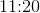? Give the smaller of the two angles.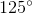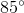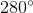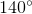Explanation:

To find the degrees of a clock hand, first find the angle between each hour-long sections. Since there areevenly spaced sections, we find that each section has an angle of: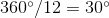. atthe hour hand has gone one-third of the way between theand. Thus there are two-thirds ofbetween the hour hand and the.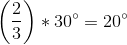.

There are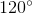betweenand, where the minute hands is. Thus there's a total of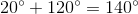between the hands.

1 3 Next →

### All ACT Math Resources